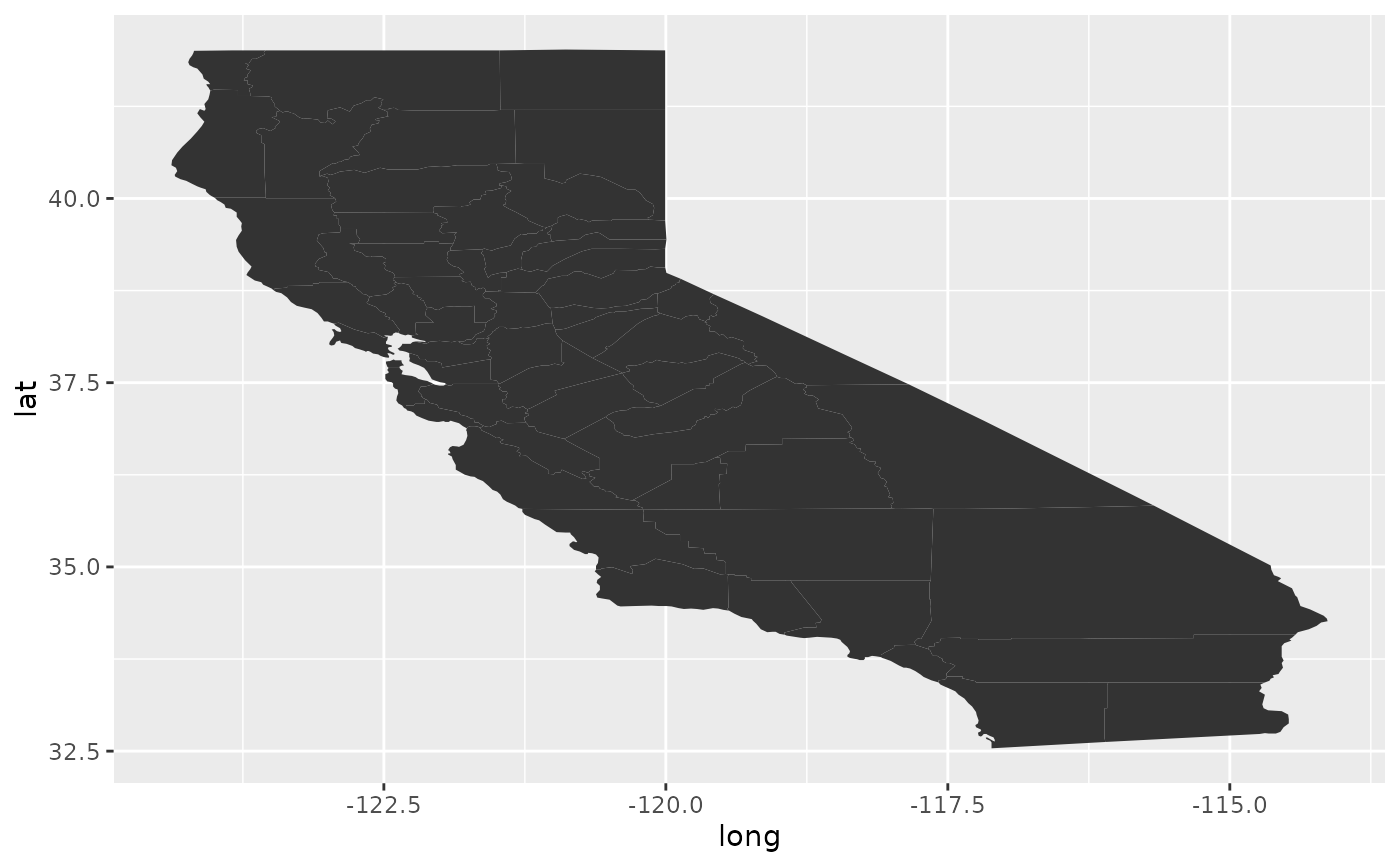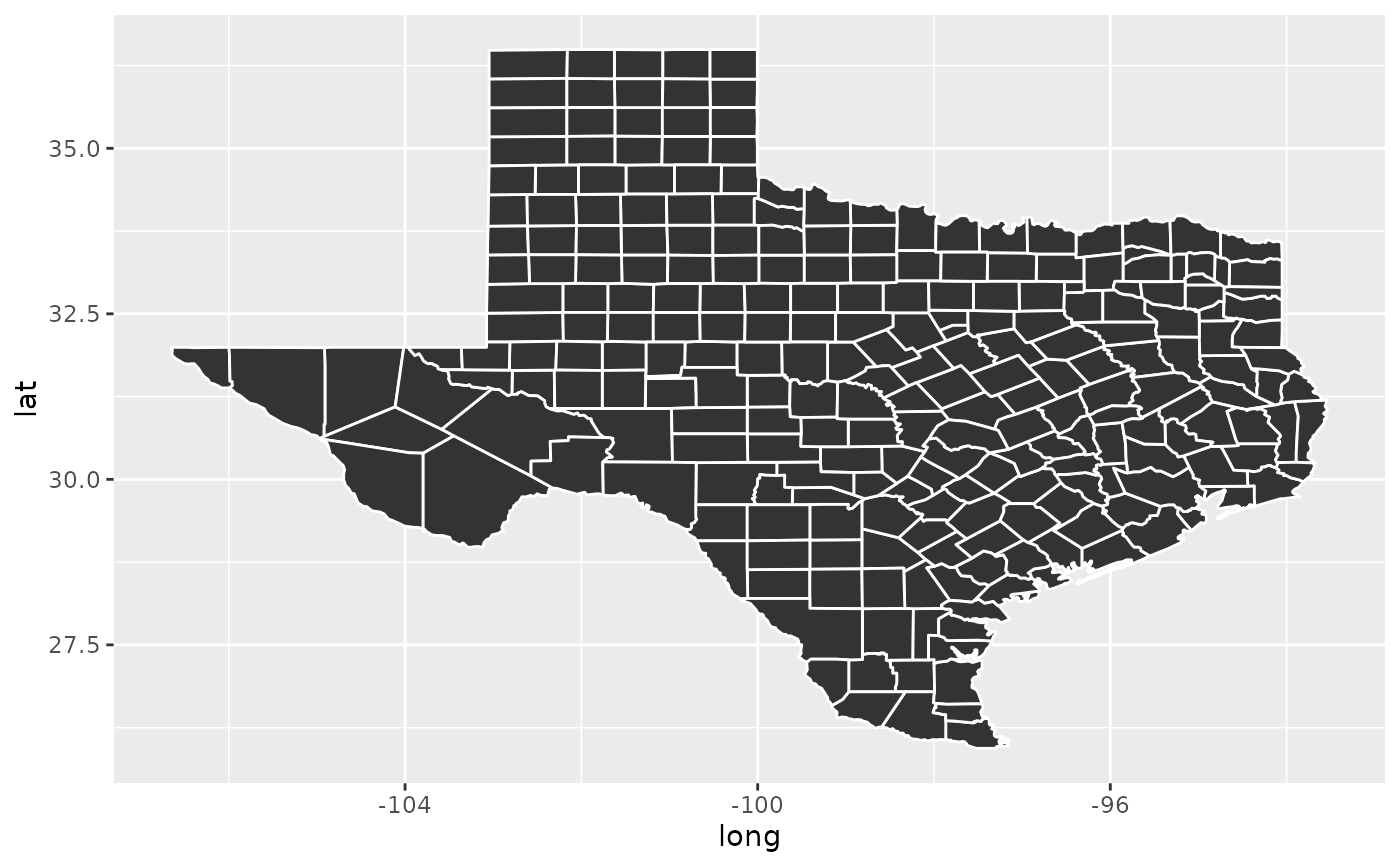This function turns a map into a data frame that can more easily be plotted with ggplot2.

# S3 method for map
fortify(model, data, ...)

## Arguments

model map object not used by this method not used by this method

## See also

map_data() and borders()

## Examples

if (require("maps")) {
ca <- map("county", "ca", plot = FALSE, fill = TRUE)
head(fortify(ca))
ggplot(ca, aes(long, lat)) +
geom_polygon(aes(group = group))
}if (require("maps")) {
tx <- map("county", "texas", plot = FALSE, fill = TRUE)
head(fortify(tx))
ggplot(tx, aes(long, lat)) +
geom_polygon(aes(group = group), colour = "white")
}